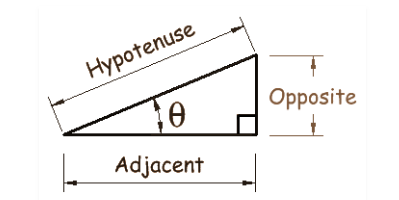# Sin Cos Formulas

Sin and Cos are basic trigonometric functions that tell about the shape of a right triangle, so the Sin Cos formulas are the basic ones in trigonometry.

• Sin A = Perpendicular/Hypotenuse
• Cos A = Base/Hypotenuse
•## Basic Trigonometric Identities for Sine and Cos

These formulas help in giving a name to each side of the right triangle and these are also used in trigonometric formulas for class 11. Let’s learn the basic sin and cos formulas.

• cos2(A) + sin2(A) = 1

If A + B = 180° then:

• sin(A) = sin(B)
• cos(A) = -cos(B)

If A + B = 90° then:

• sin(A) = cos(B)
• cos(A) = sin(B)

## Sine and Cosine Formulas

To get help in solving trigonometric functions , you need to know the trigonometry formulas.• ### Double and Triple angle formulas

• Sin 2A = 2Sin A Cos A
• Cos 2A = Cos2A – Sin2A = 2 Cos2 – 1 = 1- Sin2A
• Sin 3A = 3Sin A – 4 Sin 3A
• Cos 3A = 4 Cos3A – 3CosA
• Sin4A = 4 Cos3A . Sin A – 4Cos A. Sin 3A
• CosA = Cos4A – 6Cos2A.Sin2A +Sin4A
• Sin2A = 1Cos(2A)/2
• Cos2A =1+Cos(2A)/2

### Sum and Difference of Angles

• sin(A + B) = sin(A).cos(B) + cos(A)sin(B)
• sin(B)sin(A−B)=sin(A)⋅cos(B)−cos(A)⋅sin(B)
• cos(A+B)=cos(A)⋅cos(B)−sin(A)⋅sin(B)
• cos(A−B)=cos(A)⋅cos(B)+sin(A)⋅sin(B)
• sin(A+B+C)=sinA⋅cosB⋅cosC+cosA⋅sinB⋅cosC+cosA⋅cosB⋅sinC−sinA⋅sinB⋅sinC
• cos(A+B+C)=cosA⋅cosB⋅cosC−sinA⋅sinB⋅cosC−sinA⋅cosB⋅sinC−sinA⋅cosB⋅sinC−cosA⋅sinB⋅sinC
• Sin A + Sin B = 2Sin(A+B)/2Cos(AB)/2
• Sin A – Sin B = 2Sin(AB)/2Cos(A+B)/2
• Cos A + Cos B = 2Cos(A+B)/2Cos(AB)/2
• Cos A + Cos B = -2Sin(A+B)/2Sin(AB)/2